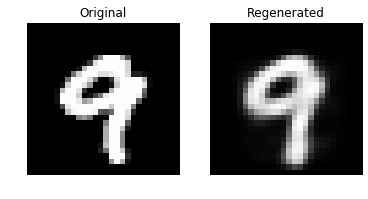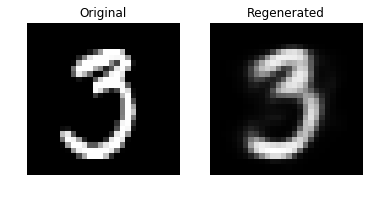# Autoencoders In Deep Learning Tutorial Using Keras

If you cut a leaf from a plant, the plant will regenerate it’s leaf once again. The plant remembers its leaf’s behavior and is able to generate the exact same leaf, If nature can then why not a machine? Fortunately, we live in a world where even a machine could do it. Today we are going to study another hot topic in deep learning, which is autoencoders. So what are we going to learn? Let’s see:-

1. Introduction
2. Architecture Of Autoencoders
3. Code overview of Autoencoders
4. Conclusion

## Introduction To Autoencoders

Autoencoders are neural network in which input data is fed to our network and output that is generated are exactly the same as the input data, not a copied data but a whole newly generated output data that has learned the features with the help of a neural network and has implemented it to regenerate from input data. Autoencoder is also a dimension reduction technique with no label associated with it, hence it comes under unsupervised learning but while the learning process is going on it is capable of generating its own labels.From the above image, we can analyze that input data is fed to a network of an encoder, code, and decoder to generate an output image. The important part of our model is to find the correlation between the input data in order to reconstruct it. It is not as simple as it looks right now, nor it is complex, but there are many steps involved in between that we will explore further, starting with the architecture of autoencoder.

## Architecture of Autoencoders

On the broader level, autoencoders are nothing but another dimensional reduction technique like principal component analysis, but a lossier one, so in order to get the output result as a reconstructed input data, we need to train our data over and over until the loss or error is less.Above is a feed-forward network that consists of an input layer, where the input weights are stored in a matrix, next step is matrix transformation, furthermore Activation function is used for linear transformation of the data. In our autoencoder architecture, we have two such ANN networks, one in the encoder and the other in the decoder. Consider your input layer with some data each, such as x1, x2, and x3 like the image below.And after the wights are introduced, the matrix transformation will be the matrix multiplication of input data and hidden layer weights as shown

below:Weights are nothing but useful information, Now consider that we extracted the features from our encoder ANN and then we compressed it using activation function, an activation function put all the incoming values in the range of -1 to 1, activation function adds some bias on incoming data in order to add non-linearity, it also acts as the decider that decides whether the incoming input data is good to go further or not.

Broadly, encoder ANN is putting some compressed input data and decoder ANN is learning from it and making an exact same replica of it, that is also the reason the output generated will always work only for that specific data and not others, For example, if you chose MNIST dataset, it will work on it specifically and not on any other random image.

## Autoencoder Keras Python code

We are going to take the MNIST dataset, it contains various numbers with different pixel values, let’s dive in. Using Keras to implement Autoencoders.

Initializing each library we need to initialize and implement our model.

``````import numpy as np
import matplotlib.pyplot as plt
import pandas as pd
from sklearn.model_selection import train_test_split

import keras
from keras.models import Model
from keras.layers import Input, Dense, Activation
from keras.utils import np_utils``````

``````dataset = pd.read_csv('../datasets/mnist_data/train.csv')
dataset = dataset.values
X, y = dataset[:, 1:]/255, dataset[:, 0]
X_train, X_test, y_train, y_test = train_test_split(X, y, test_size=0.2)
y_train[:10]``````

output : array([6, 4, 5, 9, 2, 1, 7, 3, 1, 6])

``X_train.shape, X_test.shape``

output : ((33600, 784), (8400, 784))

### Implementing AutoEncoders Keras

``````#e = 64
e = 2

inp = Input(shape=(784,))
emb = Dense(e, activation='relu')(inp)
out = Dense(784)(emb)

autoencoder = Model(inputs=inp, outputs=out)
autoencoder.summary()``````
```_________________________________________________________________
Layer (type)                 Output Shape              Param #
=================================================================
input_5 (InputLayer)         (None, 784)               0
_________________________________________________________________
dense_7 (Dense)              (None, 2)                 1570
_________________________________________________________________
dense_8 (Dense)              (None, 784)               2352
=================================================================
Total params: 3,922
Trainable params: 3,922
Non-trainable params: 0```

Above you can see the network we discussed above, we have taken the relu activation function which has adjusted quite well with our dataset, you can always play with the activation function and the number of hidden layers, you can always increase or decrease the number of hidden layers until you get satisfying results, adding more and more layers sometimes retrieve excess of information from the input that we don’t even want, and having fewer layers may not even retrieve any valuable information at all, so choose wisely.

``````autoencoder.compile(loss='mse', optimizer='adagrad', metrics=['accuracy'])
hist = autoencoder.fit(
X_train, X_train,
epochs=40,
shuffle=True,
batch_size=512,
validation_data=(X_test, X_test)
)``````

As we know, in order to train our data, we have to compare our input and output data to calculate loss, we have taken mean square error as a loss function, ‘adagrad’ is an optimizer that is based on gradient descent. We have taken 40 epochs, which shows the number of times training will be done, batch size of 512 means that a total of 512 data input is going to train at once.

``````test = X_test[:20]
preds = autoencoder.predict(test)
test.shape, preds.shape``````

output : ((20, 784), (20, 784))

``````for i in range(test.shape):
plt.figure()
plt.subplot(1,2,1)
plt.title('Original')
plt.axis('off')
plt.imshow(test[i].reshape((28,28)), cmap='gray')

plt.subplot(1,2,2)
plt.title('Regenerated')
plt.axis('off')
plt.imshow(preds[i].reshape((28,28)), cmap='gray')``````Output can be visualized using matplotlib, you can get better results by changing the number of hidden layers, batch size, or epochs.

## Conclusion

Autoencoder is a dimensionality reduction technique that has other features such as denoising the input data, auto-encoders are used in basic models, although PCA is considered to be faster than Autoencoder, the addition of non-linearity makes autoencoder unique. The autoencoder can be used as a good introductory dimensionality reduction technique in deep learning as it is easy to understand and visualize.### tanesh

Founder Of Aipoint, A very creative machine learning researcher that loves playing with the data.